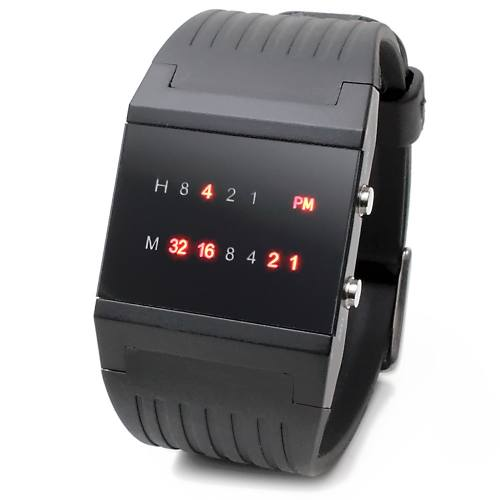### LeetCode• ㊗️
• 大家
• offer
• 多多！

## Problem

A binary watch has 4 LEDs on the top to represent the hours (0-11), and 6 LEDs on the bottom to represent the minutes (0-59). Each LED represents a zero or one, with the least significant bit on the right.

• For example, the below binary watch reads “4:51”.Given an integer turnedOn which represents the number of LEDs that are currently on (ignoring the PM), return all possible times the watch could represent. You may return the answer in any order.

The hour must not contain a leading zero.

• For example, “01:00” is not valid. It should be “1:00”. The minute must be consist of two digits and may contain a leading zero.

• For example, “10:2” is not valid. It should be “10:02”.

Example 1:

Input: turnedOn = 1
Output: ["0:01","0:02","0:04","0:08","0:16","0:32","1:00","2:00","4:00","8:00"]


Example 2:

Input: turnedOn = 9
Output: []


## Code

539. Minimum Time Difference

class Solution {
List<String> res = new ArrayList<>();

for(int h = 0; h < 12; h++){
for(int m = 0; m < 60; m++){
if(Integer.bitCount(h) + Integer.bitCount(m) == num){